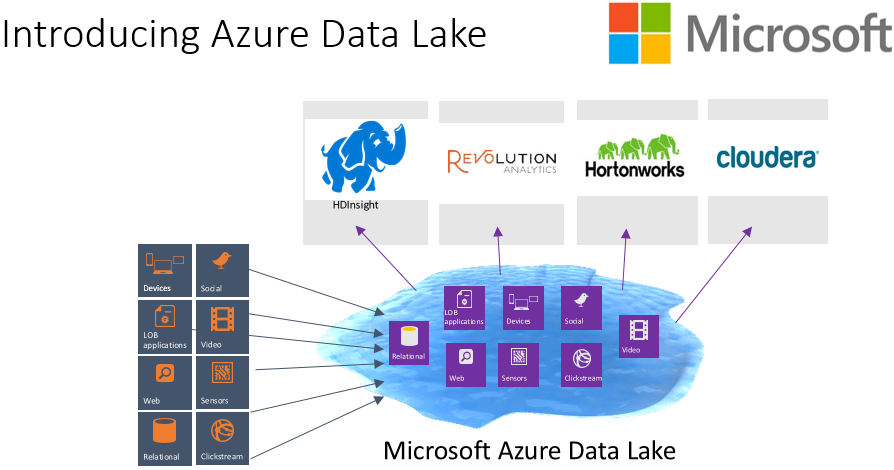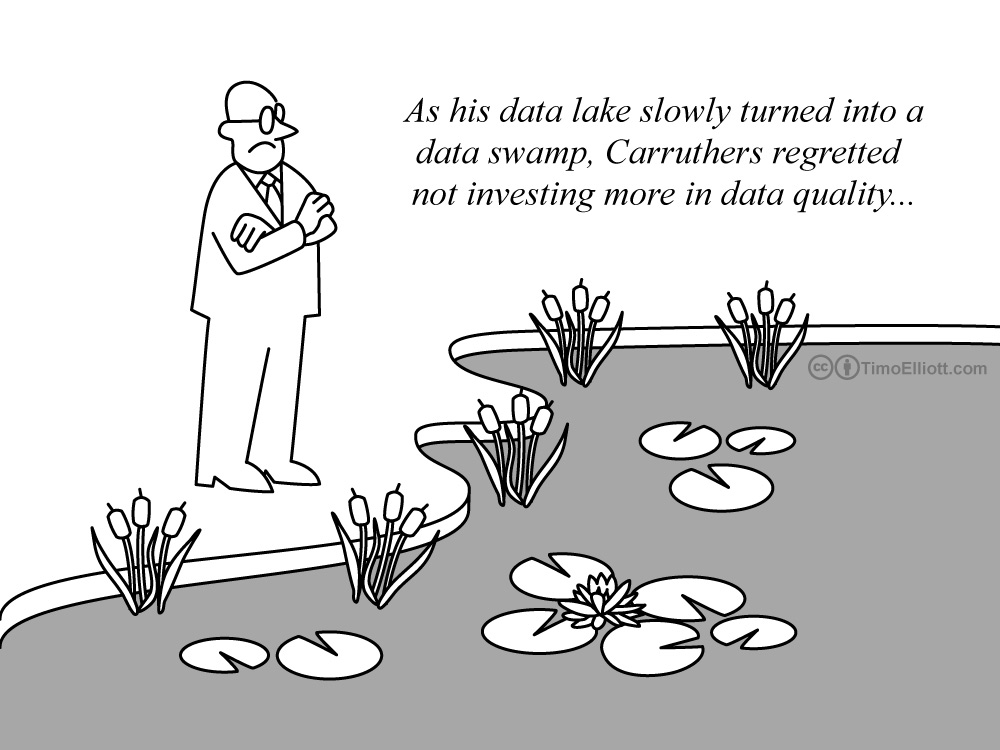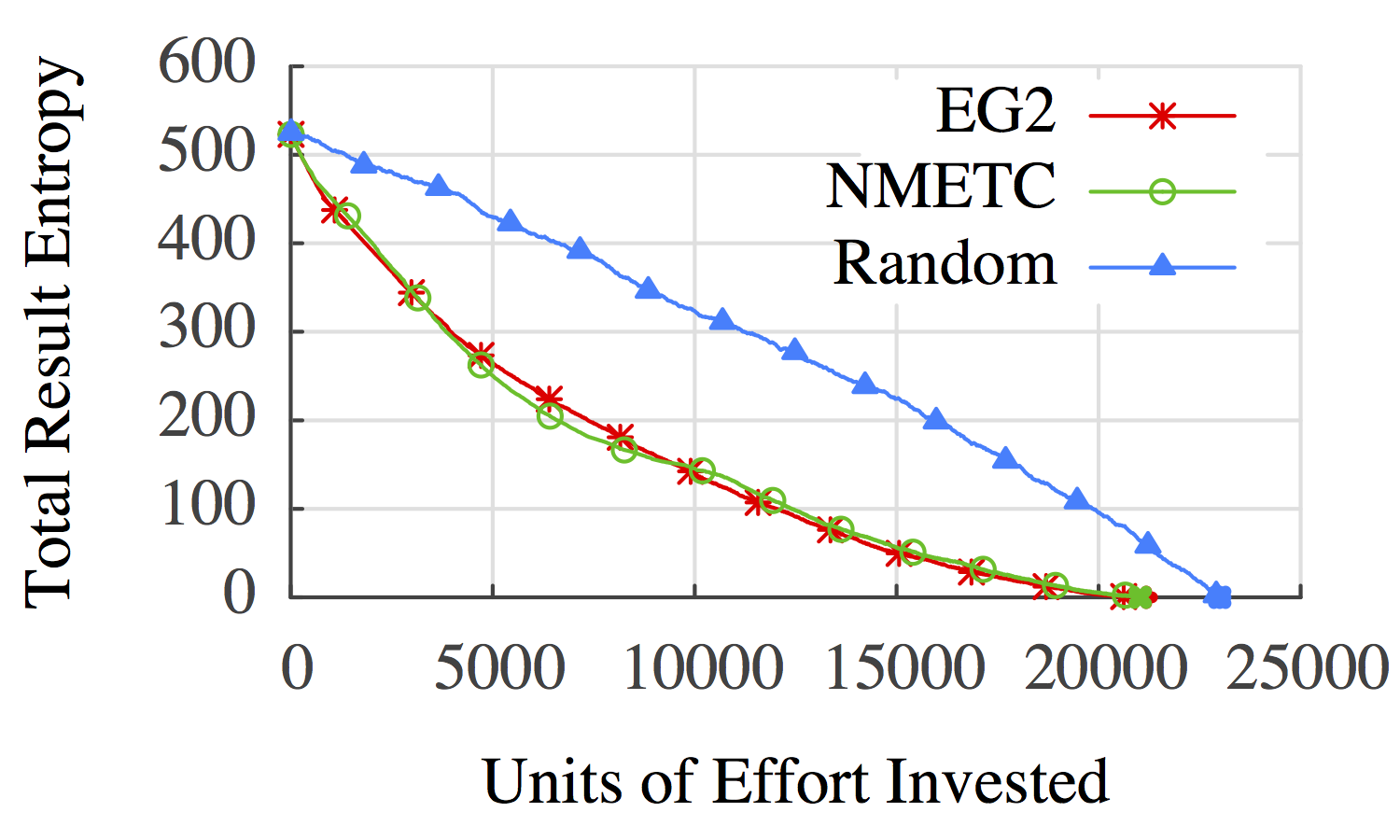Embracing Uncertainty

## Embracing Uncertainty

### A Big Data Fairy Tale#### Meet Alice

(OpenClipArt.org)#### Alice has a Store

(OpenClipArt.org)#### Alice's store collects sales data

(OpenClipArt.org)+=#### Alice wants to use her sales data to run a promotion

(OpenClipArt.org)#### So Alice loads up her sales data in her trusty database/hadoop/spark/etc... server.

(OpenClipArt.org)+ ?

#### ... asks her question ...

(OpenClipArt.org)+ ? →#### ... and basks in the limitless possibilities of big data.

(OpenClipArt.org)

## Why is this a fairy tale?## Data Cleaning is Hard!

### State of the Art(skilledup.com)

Alice spends weeks cleaning her data before using it.

### Newer State of the Art(azure.microsoft.com)(timoelliott.com)

## Making Cleaning Easier

Can we start with automation and work our way up?

### In the name of Codd,thou shalt not give the user a wrong answer.

#### What would it take for that to be ok?

• Automate educated guesses for fast cleaning
• Lenses: A family of simple data-cleaning operators
• ... but what if the guesses are wrong?
• Annotate 'best guess' relations with the guesses
• Virtual C-Tables: A lineage model based on views, labeled nulls, and lazy evaluation.
• ... so now the user needs to interpret your guesses?
• Rank guesses by their impact on result uncertainty
• CPI: A greedy heuristic for ranking sources of uncertainty.

### Lenses

Here's a problem with my data. Fix it.

• What type is this column? (majority vote)
• How do the columns of these relations line up? (pick your favorite schema matching paper)
• How do I query heterogeneous JSON objects? (see above)
• What should these missing values be? (learning-based interpolation)

### VG-Relational Algebra(Variable-Generating Relational Algebra)

• Relational Algebra
• Labeled Nulls
• Lazy Evaluation

### Labeled Nulls

$Var(\ldots)$ constructs new variables

• $Var('X')$ constructs a new variable $X$
• $Var('X', 1)$ constructs a new variable $X_{1}$
• $Var('X', ROWID)$ evaluates $ROWID$ and then constructs a new variable $X_{ROWID}$

### Lazy Evaluation

Variables can't be evaluated until they are bound.
So, we allow arbitrary expressions to be values.

• $X$ is a legitimate data value.
• $X+1$ is a legitimate data value.
• $1+1$ is a legitimate data value, but can be reduced to $2$.

A lazy value without variables is deterministic

The $Var()$ operator can be inlined into SQL


SELECT A, VAR('X', B)+2 AS C FROM R;

AB
12
34
56
AC
1$X_2+2$
3$X_4+2$
5$X_6+2$

Selects on $Var()$ need to be deferred too...


SELECT A FROM R WHERE VAR('X', B) > 2;

AB
12
34
56
A$\phi$
1$X_2>2$
3$X_4>2$
5$X_6>2$

When evaluating the table, rows where $\phi = \bot$ are dropped.

### C-Tables

• Original Formulation [Imielinski, Lipski 1981]
• PC-Tables [Green, Tannen 2006]
• Systems
• Orchestra [Green, Karvounarakis, Taylor, Biton, Ives, Tannen 2007]
• MayBMS [Huang, Antova, Koch, Olteanu 2009]
• Pip [Kennedy, Koch 2009]
• Sprout [Fink, Hogue, Olteanu, Rath 2011]
• Generalized PC-Tables [Kennedy, Koch 2009]

### Lenses

• A VG-RA Expression
• A 'Model' that defines for each variable...
• A sampling process
• A best guess estimator
• A human-readable description

Lenses implement PC-Tables


CREATE LENS PRODUCTS
AS SELECT * FROM PRODUCTS_RAW
USING DOMAIN_REPAIR(DEPARTMENT NOT NULL);

• AS clause defines source data.
• USING clause requests repairs.

CREATE LENS PRODUCTS
AS SELECT * FROM PRODUCTS_RAW
USING DOMAIN_REPAIR(DEPARTMENT NOT NULL);


#### The Query


CREATE VIEW PRODUCTS
AS SELECT ID, NAME, ...,
CASE WHEN DEPARTMENT IS NOT NULL THEN DEPARTMENT
ELSE VAR('PRODUCTS.DEPARTMENT', ROWID)
END AS DEPARTMENT
FROM PRODUCTS_RAW;

IDName...Department
123Apple 6s, White...Phone
34234Dell, Intel 4 core...Computer
34235HP, AMD 2 core...$Prod.Dept_3$
............

CREATE LENS PRODUCTS
AS SELECT * FROM PRODUCTS_RAW
USING DOMAIN_REPAIR(DEPARTMENT NOT NULL);


#### The Model


SELECT * FROM PRODUCTS_RAW;An estimator for $PRODUCTS.DEPARTMENT_{ROWID}$

## The User's View


SELECT NAME, DEPARTMENT FROM PRODUCTS;

NameDepartment
Apple 6s, WhitePhone
Dell, Intel 4 coreComputer
HP, AMD 2 coreComputer
......

Simple UI: Highlight values that are based on guesses.


SELECT NAME, DEPARTMENT FROM PRODUCTS;

NameDepartment
Apple 6s, WhitePhone
Dell, Intel 4 coreComputer
HP, AMD 2 coreComputer
......

Allow users to EXPLAIN uncertain outputs

Explanations include reasons given in English

$PRODUCTS.DEPARTMENT_{3}$

"I guessed 'Computer' for 'Department' on Row '3'"

(Generalized) C-Tables are a form of lineage.

## Selection (Filtering)


SELECT NAME FROM PRODUCTS
WHERE DEPARTMENT='PHONE'
AND ( VENDOR='APPLE'
OR PLATFORM='ANDROID' )


Recall, row-level uncertainty is a boolean formula $\phi$.

For this query, $\phi$ can be as complex as: $$DEPT_{ROWID}='P\ldots' \wedge \left( VEND_{ROWID}='Ap\ldots' \vee PLAT_{ROWID} = 'An\ldots' \right)$$

Too many variables! Which is the most important?

Data Cleaning

## Which variables are important?

The ones that keep us from knowing everything

$$D_{ROWID}='P' \wedge \left( V_{ROWID}='Ap' \vee PLAT_{ROWID} = 'An' \right)$$

$$A \wedge (B \vee C)$$

### Naive Approach

Consider a game between a database and an impartial oracle.

• The DB picks a variable $v$ in $\phi$ and pays a cost $c_v$.
• The Oracle reveals the truth value of $v$.
• The DB updates $\phi$ accordingly and repeats until $\phi$ is deterministic.

Naive Algorithm: Pick all variables!

Less Naive Algorithm: Minimize $E\left[\sum c_v\right]$.

## Exponential Time Bad!

### The Value of What We Don't Know

$$\phi = A \wedge (B \vee C)$$

1. Generate Samples for $A$, $B$, $C$
2. Estimate $p(\phi)$
3. Compute $H[\phi] = -\log\left(p(\phi) \cdot (1-p(\phi))\right)$

Entropy is intuitive:
$H = 1$ means we know nothing,
$H = 0$ means we know everything.

### Information Gain

$$\mathcal I_{A \leftarrow \top} (\phi) = H\left[\phi\right] - H\left[\phi(A \leftarrow \top)\right]$$

Information gain of $v$: The reduction in entropy from knowing the truth value of a variable $v$.

### Expected Information Gain

$$\mathcal I_{A} (\phi) = \left(p(A)\cdot \mathcal I_{A\leftarrow \top}(\phi)\right) + \left(p(\neg A)\cdot \mathcal I_{A\leftarrow \bot}(\phi)\right)$$

Expected information gain of $v$: The probability-weighted average of the information gain for $v$ and $\neg v$.

### The Cost of Perfect Information

Combine Information Gain and Cost

$$f(\mathcal I_{A}(\phi), c_A)$$

For example: $EG2(\mathcal I_{A}(\phi), c_A) = \frac{2^{\mathcal I_{A}(\phi)} - 1}{c_A}$

Greedy Algorithm: Minimize $f(\mathcal I_{A}(\phi), c_A)$ at each step

### Experimental Data

• Delete random fields (~50%).

### Experimental Queries

Simulate an analyst trying to manually explore correlations.

• Train a tree-classifier on the base data.
• Convert the decision tree to a query for all rows where the tree predicts a specific value.

### Cost vs Entropy: Credit DataEG2: Greedy Cost/Value Ordering
NMETC: Naive Minimal Expected Total Cost
Random: Completely Random Order

### Cost vs Entropy: Product DataEG2: Greedy Cost/Value Ordering
NMETC: Naive Minimal Expected Total Cost
Random: Completely Random Order

## Demo (Mimir)## Intuitive Uncertainty

UB: Ying Yang, Niccolo Meneghetti,
Arindam Nandi, Vinayak Karuppasamy

Oracle: Ronny Fehling, Zhen-Hua Liu, Dieter Gawlick